Next: 3.2.3 A Conserving Approximation Up: 3.2 Formalism Previous: 3.2.1 DCA

## 3.2.2 Multi Scale Method

The inability of a single solver within the DCA to numerically address long-ranged correlations explicitly, motivates a MSMB approach where the problem is divided further, incorporating a third, intermediate length-regime. In this approach, the lattice problem is mapped onto two clusters of different size each of which contributes correlations of length-scales up to the linear extent of their respective cluster size. The respective cluster problems are addressed using approximations adequate with the strength of the correlations on the respective scale. We choose a small DCA cluster of sizeto be solved using the QMC, thus explicitly accounting for the shortest ranged correlations in the system. Next, we invoke a second, larger cluster of size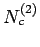to address the intermediate length regime. Except in the vicinity of phase transitions, correlations on these longer length-scales are weaker and the corresponding self-energy is approximated diagrammatically.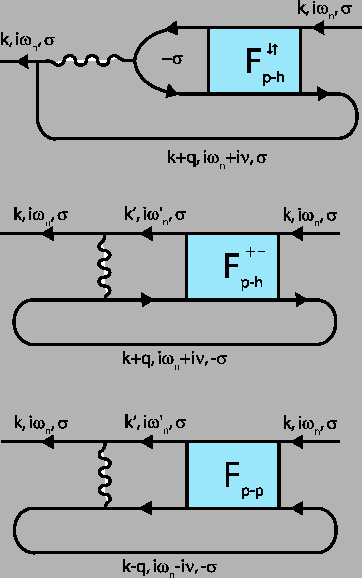We build a suitable approximation by considering the single-particle self-energy (the Hartree term is not explicitly shown) written in terms of the reducible vertexin both the particle-hole and particle-particle channel as depicted in Fig. 3.3.in turn is related to the irreducible vertexvia the Bethe-Salpeter equation (see Fig. 3.4)(3.7)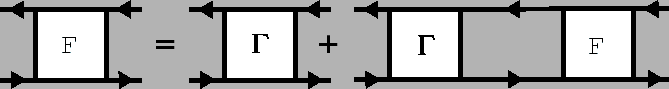In perturbation theory, the approximations to the self-energy are often made at the level of the irreducible vertex function. In the simple approximation, the resulting self-energy diagrams in Fig. 3.3 are those of the FLEX. The resulting method provides qualitatively correct long-range properties, but short ranged correlations are addressed inadequately - e.g. local moment formation.

The failure of the FLEX, and similar perturbative approaches based on the irreducible vertex, occurs in the stronger coupling regime. This is a result of neglecting higher order correction terms which re-normalize the vertex. In a non-perturbative approach, Y.M. Vilk et.al. in Ref. (10) considered a renormalized static vertex to address this problem.

In the MSMB method however, we introduce the following non-perturbative approximation for the intermediate length-regime: The large cluster irreducible two-particle vertex is approximated by the small cluster irreducible vertex thus preserving the exact short ranged correlation (on the small cluster) and approximating the intermediate ranged ones (on the large cluster):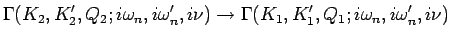(3.8)

where momenta labeled with subscript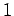are cluster momenta on the small cluster, while subscript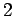denotes large cluster momenta. The approximated self-energy on the large cluster is evaluated from the Dyson equation depicted in Fig. 3.3. It preserves all short ranged correlations and includes long-wave length contributions which emerge from the inclusion of large cluster corrections through the bare bubble, i.e. the reducible part of the vertex. However, by implementing the DCA on a two-particle irreducible level (i.e. replacing the large cluster irreducible vertex with the small cluster equivalent), the resulting self-energy is inherently different from the single-particle DCA self-energy that one would obtain from the usual, direct DCA/QMC simulation of the large cluster (see Sec. 3.2.1). Only in the case of infinite dimensions, where the problem becomes purely local, are these two approaches equivalent.

A few remarks about the above approximation are in order. The approximation in Eq. 3.8 breaks the crossing symmetry of(as it is related toby Eq. 3.7), sincenow contains long-ranged corrections beyond the linear extent of the small cluster only in the channel it is calculated in. Hence, the self-energy contribution have to be evaluated in all three depicted channels (see Fig. 3.3). Furthermore, this MSMB approach requires a full knowledge of the momentum and frequency exchange on the cluster and hence the evaluation ofon the small cluster still involves extensive numerical calculations and is limited by storage/memory requirements (see Sec. 3.6 for details).

This difficulty of obtaining the small cluster irreducible vertex, necessitates a further long-ranged approximation for. In ref. (13) Abrikosov et. al. have, in their study of Fermi liquid theory, identified a sub-class of self-energy diagrams which convey the long length-scale properties of a system. These are constructed from irreducible vertex functions with zero external momentum and frequency transfer. In applying this restriction we expect to capture long ranged correlations which are characterized by small momentum transfers. The resulting long wave-length approximated irreducible vertex, denoted by the index, is given by(3.9)

The MSMB approximation at the level of the irreducible vertex is expected to provide the best description for the most dominant two-particle processes. Furthermore, any phase transitions would manifest themselves in instabilities of the reducible vertex which contains the singular structure while the irreducible vertex would remain analytic throughout. However, the resulting fluctuation diagram representation (Fig. 3.3) is expected to remain most valid away from phase transitions where intermediately ranged correlations are weak. When these correlations are not weak, a fluctuation representation of the self energy may contain significant numerical errors, but such an approach can nonetheless represent non-trivial aspects of strong correlations such as non-Fermi-liquid behavior(14,15). Therefore, the infinite order MSMB approximation is expected to at least qualitatively represent the emergence of charge and/or spin order in lower dimensional systems. Further details on the calculation of theself-energy are provided in section 3.3.

The self-energy obtained in this-approximation only correctly accounts for long-ranged fluctuations of the system. The remaining short length-scale contributions have been neglected and have to be accounted for separately as will be discussed in a subsequent section 3.2.4.Next: 3.2.3 A Conserving Approximation Up: 3.2 Formalism Previous: 3.2.1 DCA
© Cyrill Slezak